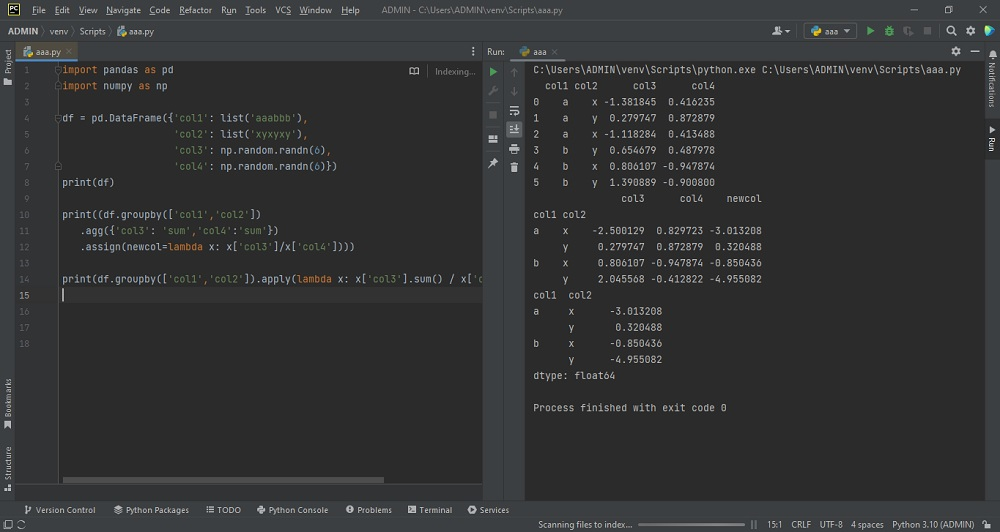# Summarize using multiple columns in python pandas dataframeby vsasikalabeUpdated: Feb 28, 2023Solution Kit

The ability to summarize data using multiple columns in Pandas DataFrame is an essential technique for real-world data analysis. Here are a few ways this technique can be helpful: Grouping and summarizing data by multiple columns allows us to explore relationships between different variables. For example, in market research, we can group data by multiple columns, such as age and gender, and then calculate summary statistics, such as each group's average income or spending. This can help us identify patterns and trends in consumer behavior and preferences.

A lambda function is one of the most flexible ways to apply custom aggregation functions. A lambda function is a tiny, anonymous function that can take any number of arguments and only have a single expression. We can use lambda functions with groupby() to define custom aggregation functions specific to our needs. In Pandas, groupby() is a powerful method that allows us to group a DataFrame by one or more columns and apply an aggregation function to each group.

The ability to summarize data using multiple columns in Pandas DataFrame is a powerful technique that can help us gain insights, identify patterns, and make data-driven decisions in various real-world applications.

Here is an example of how to Summarize using multiple columns in the python pandas dataframe:Preview of the output that you will get on running this code from your IDE.

### Code

In this solution we used pandas and numpy library of python.

### Instructions

Follow the steps carefully to get the output easily.

2. Install pandas on your IDE from python interpreter in setting options.
3. Create new python file on your IDE.
4. Copy the snippet using the 'copy' button and paste (don't take the out statements) it in your python file.
5. import the pandas and numpy library.Add print statement to the end.
6. Run the current file to generate the output.

I hope you found this useful. I have added the link to dependent library, version information in the following sections.

I found this code snippet by searching for ' Summarize using multiple columns in python pandas dataframe ' in kandi. You can try any such use case!

### Environment Tested

I tested this solution in the following versions. Be mindful of changes when working with other versions.

1. PyCharm Community Edition 2022.3.1
2. The solution is created in Python 3.11.1 Version
3. pandas 1.5.2 Version
4. numpy 1.24.1 version

Using this solution, we can do Summarize using multiple columns in python pandas dataframe.This process also facilities an easy to use, hassle free method to create a hands-on working version of code in python which would help us to do Summarize using multiple columns in python pandas dataframe.

### Dependent Libraries

pandasby pandas-dev

Python38499Version:v2.0.2Flexible and powerful data analysis / manipulation library for Python, providing labeled data structures similar to R data.frame objects, statistical functions, and much more

Support
Quality
Security
Reuse

pandasby pandas-dev

Python38499Version:v2.0.2License: Permissive (BSD-3-Clause)

Flexible and powerful data analysis / manipulation library for Python, providing labeled data structures similar to R data.frame objects, statistical functions, and much more
Support
Quality
Security
Reuse

numpyby numpy

Python23587Version:v1.24.3The fundamental package for scientific computing with Python.

Support
Quality
Security
Reuse

numpyby numpy

Python23587Version:v1.24.3License: Permissive (BSD-3-Clause)

The fundamental package for scientific computing with Python.
Support
Quality
Security